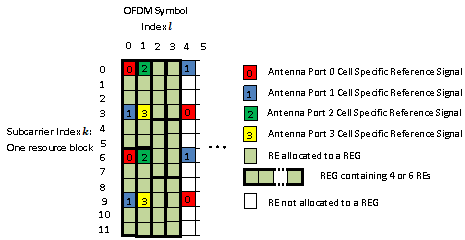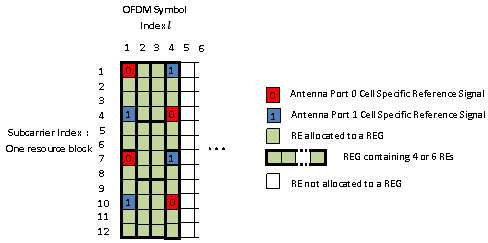Documentation

## Resource Element Groups (REGs)

Resource-element groups (REG) are used to define the mapping of control channels to resource elements (RE).

REGs are blocks of consecutive REs within the same OFDM symbol. The REGs within a subframe are located in the first four OFDM symbols and are identical in size and number for each corresponding subframe on every antenna port.

### Resource Element Group Indexing

REGs are represented by an index pair $\left({k}^{\prime },{l}^{\prime }\right)$. The index ${k}^{\prime }$ is the subcarrier index of the RE within the REG with the lowest subcarrier index k. The index ${l}^{\prime }$ is the OFDM symbol index of the REG (l). This index pair is illustrated in the following figure.### Size and Location of REGs

The number of REs within a REG is such that a REG contains four REs which are not occupied by a cell specific reference signal on any antenna port in use.

All REs within a resource block in one of the first four OFDM symbols are allocated to a REG. Therefore the number of REs within each REG and the number of REGs within an OFDM symbol is affected by the number of cell-specific reference signals present on all antenna ports.

The number and location of cell specific reference signals are dependent on the number of antenna ports and the type of cyclic prefix used.

### Antenna Port Configurations

Each antenna port has a unique cell specific reference signal associated with it. As the REG arrangement is affected by cell specific reference signals, the REG arrangement for a one or two antenna port configuration or four antenna port configuration is different. The REG arrangement for each resource block within a subframe and for every antenna port is identical.

### REG Arrangement with a Normal Cyclic Prefix

The REG arrangement for each antenna port configuration is described below for a normal cyclic prefix.

#### One or Two Antenna Port Configuration

When antenna port 0 or ports 0 and 1 are used it is assumed the cell specific reference signal is present on both antenna ports 0 and 1. This leads to a REG arrangement for each resource block as shown in the following figure.Cell-specific reference signals are present within the first OFDM symbol. As four REs not containing cell specific reference signals are required in a REG, the twelve REs in the first symbol are divided into two REGs, each containing six REs (two containing cell specific reference signals and four empty). In the second and third OFDM symbols no cell specific reference signal is present therefore the twelve REs in each symbol are divided between three REGs, each containing four REs.

#### Four Antenna Port Configuration

The REG arrangement in each resource block for four antenna port configuration is shown in the following figure.The REG allocation within the first OFDM symbol is the same as for a one or two antenna port configuration. Four cell specific reference signals are present in the second OFDM symbol therefore eight REs are available for the mapping of control data. The twelve REs are divided into two REGs, each containing six REs. The third and fourth OFDM symbols contain no reference signals so three REGs are available.

### REG Arrangement with an Extended Cyclic Prefix

An extended cyclic prefix subframe contains twelve OFDM symbols as opposed to fourteen for a normal cyclic prefix. As the number of cell specific reference signals in a normal or extended cyclic prefix subframe is the same, the limited number of OFDM symbols in an extended cyclic prefix subframe requires the OFDM symbol spacing of the cell specific reference signals to be reduced compared to when using a standard cyclic prefix.

This reduction is spacing causes cell specific reference signals to be present within the fourth OFDM symbol of an extended cyclic prefix subframe whilst in a normal cyclic prefix subframe no cell specific reference signals are present. Therefore when an extended cyclic prefix is used two REGs, each containing six REs, are present in the fourth OFDM symbol.

The number of cell specific reference symbols within the first three OFDM symbols is identical for normal or extended cyclic prefix therefore the REG configurations are identical.

#### One or Two Antenna Port Configuration

The REG arrangement for a one or two antenna port configuration when using an extended cyclic prefix is shown in the following figure.#### Four Antenna Port Configuration

The REG arrangement for a four antenna port configuration when using an extended cyclic prefix is shown in the following figure.